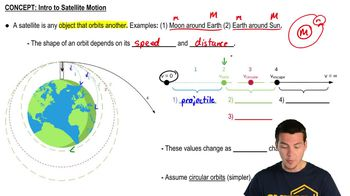Start typing, then use the up and down arrows to select an option from the list.
5:02 minutes
Problem 13c
Textbook Question

# Two satellites are in circular orbits around a planet that has radius 9.00 * 10^6 m. One satellite has mass 68.0 kg, orbital radius 7.00 * 10^7 m, and orbital speed 4800 m/s. The second satellite has mass 84.0 kg and orbital radius 3.00 * 10^7 m. What is the orbital speed of this second satellite?Verified Solution
This video solution was recommended by our tutors as helpful for the problem above.
90views